# N-Dimensional Real Division Algebras

Gold Member
I am reading Matej Bresar's book, "Introduction to Noncommutative Algebra" and am currently focussed on Chapter 1: Finite Dimensional Division Algebras ... ...

I need help with understanding some remarks that Matej Bresar makes in Chapter 1 ...

The relevant text is as follows: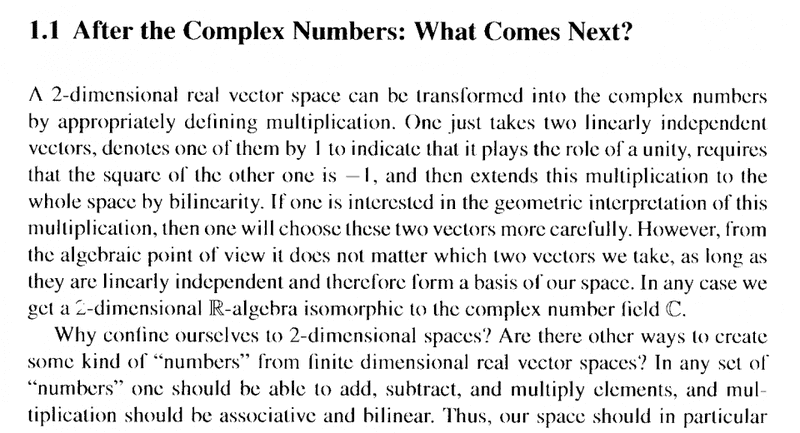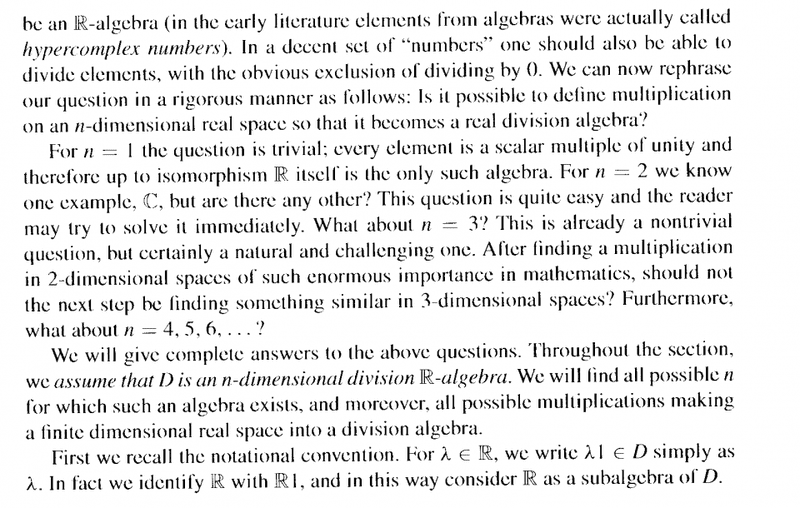My questions regarding the above text are as follows:

Question 1

In the above text from Bresar we read the following:

" ... ... Is it possible to define multiplication on an ##n##-dimensional real space so that it becomes a real division algebra?

For n = 1 the question is trivial; every element is a scalar multiple of unity and therefore up to an isomorphism ##\mathbb{R}## itself is the only such algebra. ... ... "

How do we know exactly (rigorously and formally) that up to an isomorphism ##\mathbb{R}## itself is the only such algebra?

Question 2

In the above text from Bresar we read the following:

" ... ... for ##n = 2## we know one example, ##\mathbb{C}##, but are there any other? This question is quite easy and the reader may try to solve it immediately. ... ... "

Can someone please help me to answer the above question posed by Bresar?

Question 3

In the above text from Bresar we read the following:

" ... ... what about ##n = 3##? ... ... "

Bresar answers this question on page 4 after proving Lemmas 1.1, 1.2, and 1.3 ... (see uploads below)

Bresar writes:

" ... ... Lemma 1.3 rules out the case where ##n = 3##. ... ... "

Can someone please help me to understand why/how Lemma 1.3 rules out the case where ##n = 3##?

Lemma 1.3 and its proof read as follows: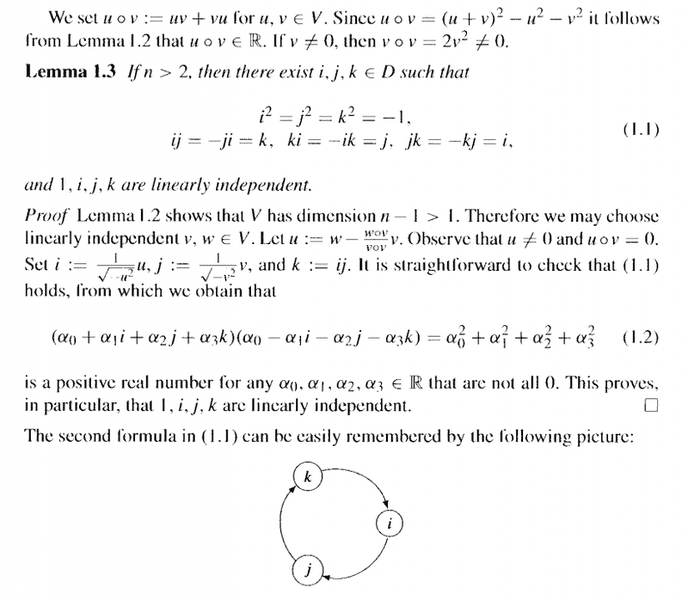Help with the above questions will be much appreciated ... ...

Peter

============================================================================

So that readers of the above post can reference other parts of Bresar's arguments, Lemmas and proofs ... as well as appreciate the context of my questions I am providing pages 1-4 of Matej Bresar's book ... as follows: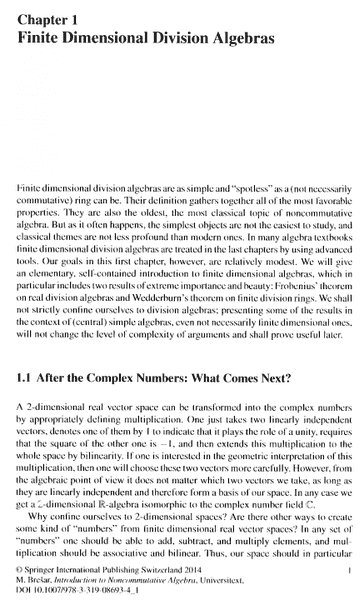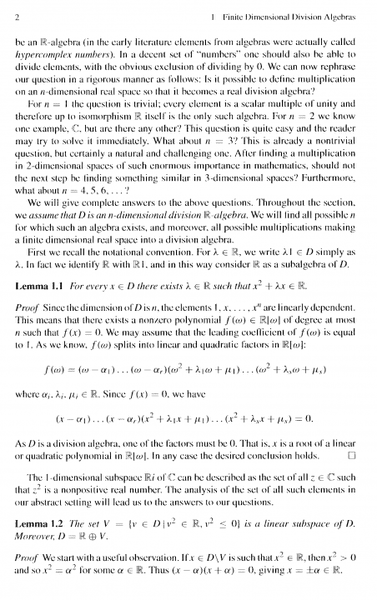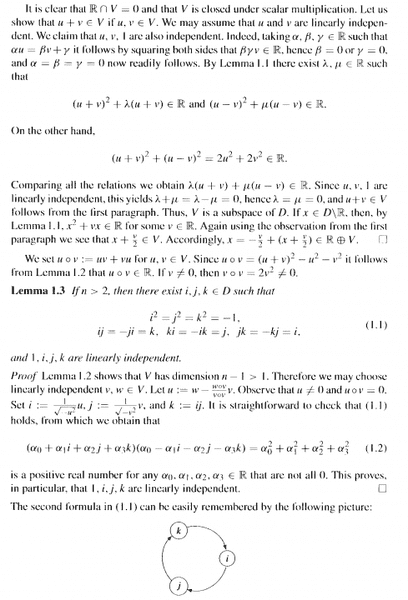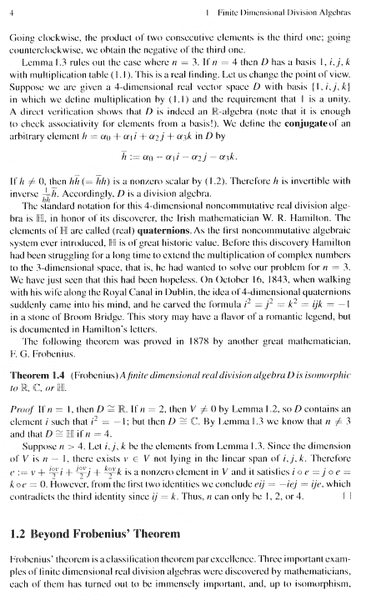## Answers and Replies

fresh_42
Mentor
As to questions one and two, it's as so often: rigor lies in the precision of wording.

The division algebras ##D## are defined as those "containing" ##\mathbb{R}## (cp. discussion in a previous thread).
To be more precise: division algebras here are real vector spaces, i.e. ##\forall_{r\in \mathbb{R}}\; r\cdot 1_D \in D##. This means a copy of ##\mathbb{R}## is via the mapping ##r \leftrightarrows r\cdot 1_D## a one-dimensional subspace of ##D##. Since ##\dim D = 1## it is already equal.

Of course this mapping is an isomorphism and it is the reason why @andrewkirk spoke of ##\mathbb{R}'## instead of ##\mathbb{R}## in https://www.physicsforums.com/threads/bresar-lemma-1-2-finite-division-algebras.894043/#post-5625075

As to question 3: In Lemma 1.3. we have already four ##\mathbb{R}-##linear independent elements ##1,i,j,k \in D## if ##n>2##. So ##n>2## implies that even ##n\geq 4## holds.

I suggest you work out the case of dimension two as an exercise. You may use Lemmata 1.1.,1.2.,1.3. and the definition of ##V##. ##\mathbb{C}## is already an example. One has to show that any other ##2-##dimensional division algebra over the reals look like it, i.e. has an element ##j## with ##j^2=-1## that can be mapped to the imaginary ##i## in ##\mathbb{C}##.
The main goal here is again to be precise. Students (and not only them) often hide uncertainties by the use of many unnecessary words, in an attempt that truth will be miraculously hidden somewhere if it only were long enough. Guess I've already beaten myself, since this gets definitely too long ...

andrewkirk
Science Advisor
Homework Helper
Gold Member
How do we know exactly (rigorously and formally) that up to an isomorphism ##\mathbb{R}## itself is the only such algebra?
Let another 1D division algebra be V. Consider the map ##f:\mathbb R\to V## that maps 0 to ##0_V##, 1 to ##1_V## and is then extended linearly to be a linear map between vector spaces. It is a result of linear algebra that ##f## is a vector space isomorphism.

We only need to prove that ##f(r\times s)=f(r)\times_Vf(s)##, since the rest of the necessary properties are obtained from the fact that it is a vector space isomorphism.

See if you can do that, using the fact that ##r=r\cdot 1,s=s\cdot 1## and that the vector space homomorphism property gives us that ##f(r\cdot 1)=r\cdot f(1)##. You will need to use the 'compatibility with scalars' axiom for V, as set out here.

In the above I am using ##\times## or ##\times_V## to indicate multiplication of vectors and ##\cdot## to indicate left-multiplication of a vector by a scalar.

•Math Amateur
Stephen Tashi
Science Advisor
Just out of curiosity - the results of lemma 1.1 depend on factoring a polynomial in ##\mathbb{R}[\omega]##, so what happens when we study finite dimensional division algebras over ##\mathbb{Q}## instead of ##\mathbb{R}## ?

fresh_42
Mentor
Just out of curiosity - the results of lemma 1.1 depend on factoring a polynomial in ##\mathbb{R}[\omega]##, so what happens when we study finite dimensional division algebras over ##\mathbb{Q}## instead of ##\mathbb{R}## ?
It will get a little bit messy, because every field extension of ##\mathbb{Q}## is a division algebra, ##\mathbb{Q}(\sqrt{83},i)## an algebraic extension, ##\mathbb{Q}(\pi, e, 2^{\sqrt{2}})## a transcendental. Plus the same division algebras as over ##\mathbb{R}##, only with ##\mathbb{Q}##.

Edit: Cancel the transcendental.

Stephen Tashi
Science Advisor
every field extension of ##\mathbb{Q}## is a division algebra

It hard to understand that phenomena intuitively. Why does less capability in factoring ( by ##\mathbb{Q}[\omega_1, \omega_2,...] ## vis-a-vis ##\mathbb{R}[\omega]## lead to more versatility in the possible finite dimensional division algebras ?

Here, I'm thinking of ##\mathbb{Q}[\omega]## and ##\mathbb{R}[\omega] ##as being polynomials involving the "indeterminate" ##\omega##, not as polynomials in some specific mathematical object like ##\omega = \sqrt{3}##.

andrewkirk
Science Advisor
Homework Helper
Gold Member
I suppose it relates to the fact that there is more diversity in the types of factors that irreducible polynomials over ##\mathbb Q## can have. Over ##\mathbb R## the factor polynomials can have order only 1 or 2, whereas over ##\mathbb Q## they can have any order.

Less capability in factoring goes along with a richer variety of types of factors.

At the extreme, in ##\mathbb C## all factors are the same type: ##(x-z_k)##.

•Stephen Tashi